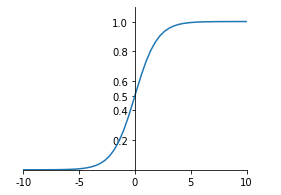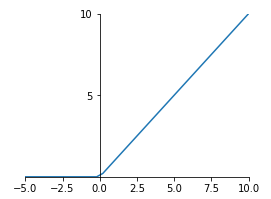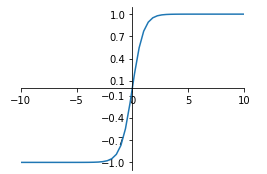# 1 什么是激活函数¶

$$y = Activation(\sum\limits_i^N {{w_i} \cdot {x_i} + b} )$$

# 2.1 sigmoid函数¶

sigmoid函数可以将整个实数范围的的任意值映射到[0,1]范围内，当当输入值较大时,sigmoid将返回一个接近于1的值,而当输入值较小时,返回值将接近于0。sigmoid函数数学公式和函数图像如下所示：

$$f(x) = \frac{1}{{1 + {e^{ - x}}}}$$In :
import tensorflow as tf
x = tf.linspace(-5., 5.,6)
x

Out:
<tf.Tensor: id=3, shape=(6,), dtype=float32, numpy=array([-5., -3., -1.,  1.,  3.,  5.], dtype=float32)>

In :
tf.keras.activations.sigmoid(x)

Out:
<tf.Tensor: id=4, shape=(6,), dtype=float32, numpy=
array([0.00669285, 0.04742587, 0.26894143, 0.7310586 , 0.95257413,
0.9933072 ], dtype=float32)>
In :
tf.sigmoid(x)

Out:
<tf.Tensor: id=5, shape=(6,), dtype=float32, numpy=
array([0.00669285, 0.04742587, 0.26894143, 0.7310586 , 0.95257413,
0.9933072 ], dtype=float32)>

sigmoid优缺点总结：

• 优点：输出的映射区间(0,1)内单调连续，非常适合用作输出层，并且比较容易求导。

• 缺点：具有软饱和性，即当输入x趋向于无穷的时候，它的导数会趋于0，导致很容易产生梯度消失。

# 2.2 relu函数¶

Relu（Rectified Linear Units修正线性单元），是目前被使用最为频繁得激活函数，relu函数在x<0时，输出始终为0。由于x>0时，relu函数的导数为1，即保持输出为x，所以relu函数能够在x>0时保持梯度不断衰减，从而缓解梯度消失的问题，还能加快收敛速度，还能是神经网络具有稀疏性表达能力，这也是relu激活函数能够被使用在深层神经网络中的原因。由于当x<0时，relu函数的导数为0，导致对应的权重无法更新，这样的神经元被称为"神经元死亡"。

relu函数公式和图像如下：

$$f(x) = \max (0,x)$$tf.keras.activations.relu( x, alpha=0.0, max_value=None, threshold=0 )

• x：输入的变量
• alpha：上图中左半边部分图像的斜率，也就是x值为负数（准确说应该是小于threshold）部分的斜率，默认为0
• max_value：最大值，当x大于max_value时，输出值为max_value
• threshold：起始点，也就是上面图中拐点处x轴的值
In :
x = tf.linspace(-5., 5.,6)
x

Out:
<tf.Tensor: id=9, shape=(6,), dtype=float32, numpy=array([-5., -3., -1.,  1.,  3.,  5.], dtype=float32)>
In :
tf.keras.activations.relu(x)

Out:
<tf.Tensor: id=10, shape=(6,), dtype=float32, numpy=array([0., 0., 0., 1., 3., 5.], dtype=float32)>
In :
tf.keras.activations.relu(x,alpha=2.)

Out:
<tf.Tensor: id=11, shape=(6,), dtype=float32, numpy=array([-10.,  -6.,  -2.,   1.,   3.,   5.], dtype=float32)>
In :
tf.keras.activations.relu(x,max_value=2.)  # 大于2部分都将输出为2.

Out:
<tf.Tensor: id=16, shape=(6,), dtype=float32, numpy=array([0., 0., 0., 1., 2., 2.], dtype=float32)>
In :
tf.keras.activations.relu(x,alpha=2., threshold=3.5)  # 小于3.5的值按照alpha * (x - threshold)计算

Out:
<tf.Tensor: id=27, shape=(6,), dtype=float32, numpy=array([-17., -13.,  -9.,  -5.,  -1.,   5.], dtype=float32)>

# 2.3 softmax函数¶

softmax函数是sigmoid函数的进化，在处理分类问题是很方便，它可以将所有输出映射到成概率的形式，即值在[0,1]范围且总和为1。例如输出变量为[1.5,4.4,2.0]，经过softmax函数激活后，输出为[0.04802413, 0.87279755, 0.0791784 ],分别对应属于1、2、3类的概率。softmax函数数学公式如下：

$$f({x_i}) = \frac{{{e^{{x_i}}}}}{{\sum\limits_i {{e^{{x_i}}}} }}$$
In :
tf.nn.softmax(tf.constant([[1.5,4.4,2.0]]))

Out:
<tf.Tensor: id=29, shape=(1, 3), dtype=float32, numpy=array([[0.04802413, 0.87279755, 0.0791784 ]], dtype=float32)>
In :
tf.keras.activations.softmax(tf.constant([[1.5,4.4,2.0]]))

Out:
<tf.Tensor: id=31, shape=(1, 3), dtype=float32, numpy=array([[0.04802413, 0.87279755, 0.0791784 ]], dtype=float32)>
In :
x = tf.random.uniform([1,5],minval=-2,maxval=2)
x

Out:
<tf.Tensor: id=38, shape=(1, 5), dtype=float32, numpy=
array([[ 1.9715171 ,  0.49954653, -0.37836075,  1.6178164 ,  0.80509186]],
dtype=float32)>
In :
tf.keras.activations.softmax(x)

Out:
<tf.Tensor: id=39, shape=(1, 5), dtype=float32, numpy=
array([[0.42763966, 0.09813169, 0.04078862, 0.30023944, 0.13320053]],
dtype=float32)>

# 2.4 tanh函数¶

tanh函数无论是功能还是函数图像上斗鱼sigmoid函数十分相似，所以两者的优缺点也一样，区别在于tanh函数将值映射到[-1,1]范围，其数学公式和函数图像如下：

$$f(x) = \frac{{\sinh x}}{{\cosh x}} = \frac{{1 - {e^{ - 2x}}}}{{1 + {e^{ - 2x}}}}$$In :
x = tf.linspace(-5., 5.,6)
x

Out:
<tf.Tensor: id=43, shape=(6,), dtype=float32, numpy=array([-5., -3., -1.,  1.,  3.,  5.], dtype=float32)>
In :
tf.keras.activations.tanh(x)

Out:
<tf.Tensor: id=44, shape=(6,), dtype=float32, numpy=
array([-0.99990916, -0.9950547 , -0.7615942 ,  0.7615942 ,  0.9950547 ,
0.99990916], dtype=float32)>

# 3 总结¶

posted @ 2019-10-21 08:03 奥辰 阅读(...) 评论(...) 编辑 收藏